# 10-327/Solution to Almost Disjoint Subsets

I think that this collection satisfies the properties.

Let$A$ be the set of all infinite sequences of 0's and 1's. Let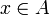$x \in A$ and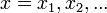$x=x_1,x_2,...$ with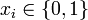$x_i \in \{0,1\}$ Let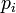$p_i$ be the ith prime number i.e.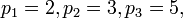$p_1 = 2, p_2=3, p_3=5,$ etc.

Let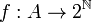$f:A \to 2^{\mathbb{N}}$

Such that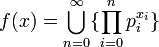$f(x)=\bigcup_{n=0}^{\infty}\{\prod_{i=0}^n p_i^{x_i}\}$

ie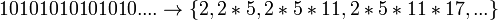$10101010101010.... \to \{2,2*5,2*5*11,2*5*11*17,...\}$

Then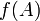$f(A)$ is a collection of sets with the desired properties (I think).

• I don't have time to write out the whole proof, and haven't gone over it completely yet but it seems to work. Showing the the function is injective gives uncountablity. And proving that if they have an infinite intersection they have the same preimage, which is just a single point by injective, they are the same set. - John
• Your solution works, though there are simpler solutions. Drorbn 20:57, 19 October 2010 (EDT)